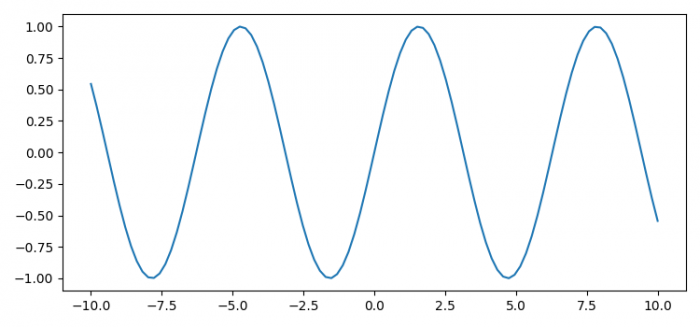# How to have a function return a figure in Python (using Matplotlib)?

To have a function return a figure in Python (using Matplotlib), we can take the following steps −

• Set the figure size and adjust the padding between and around the subplots.

• Create x and y data points using numpy.

• Make a function plot(x, y) that creates a new figure or activate an existing figure using figure() method.

• Plot the x and y data points using plot() method; return fig instance.

• Call plot(x, y) method and store the figure instance in a variable, f.

• To display the figure, use show() method.

## Example

import numpy as np
from matplotlib import pyplot as plt

plt.rcParams["figure.figsize"] = [7.50, 3.50]
plt.rcParams["figure.autolayout"] = True

def plot(x, y):
fig = plt.figure()
plt.plot(x, y)
return fig

x = np.linspace(-10, 10, 100)
y = np.sin(x)

f = plot(x, y)

plt.show()

## Output